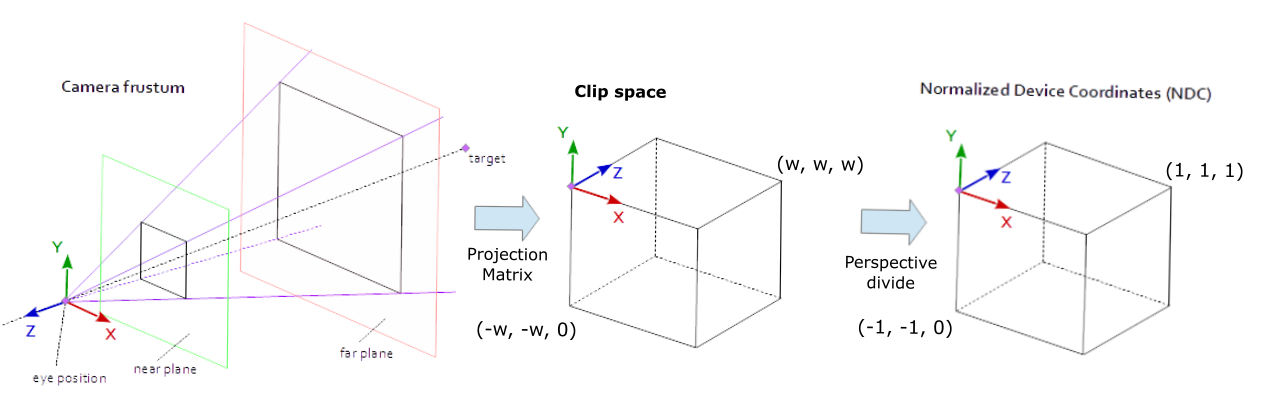# How project(camera) work in threejs?

I used this code `

``````new THREE.Vector3(0,5,2).project(camera)
``````

got this result
n {x: 0, y: 2.1720422880686763, z: 0.9335266860019336}
when doing

``````new THREE.Vector3(0,5,2).unproject(camera)
``````

got this :n {x: -0.29274196547907116, y: 11.222416135936928, z: 16.674224015138016}
How this works which mathematic formula used to get this result .

I used Thales Theorem formula using this figure
figure
but not getting the required resultBoth `THREE.Vector3.project` and `THREE.Vector3.unproject` perform calculation by multiplication of the vector by two matrices.

Some of the fundamental mathematical operations in Computer Graphics are matrix calculations. A lot of transformations can be represented and processed by matrices – translation, rotation, scaling, mirroring, switching coordinate systems, … and projection.

In Three.js `THREE.Vector3.project` calculates the normalized coordinates of a vertex. These coordinates are in the range [-1,1]. As expected this is done by two matrix multiplications – one matrix that encodes the 3D position and orientation of the camera; and a second matrix that encodes the intrinsic properties of the camera that define the size and shape of its frustum.

The opposite operation is `THREE.Vector3.unproject` that calculates the frustum coordinates of a vector in normalized coordinates. Naturally it is done by two matrix multiplications too – the inverse matrices from the projection, but multiplied in reverse order.

Look what it says here:

Projects this vector from world space into the camera’s normalized device coordinate (NDC) space.

I know you did not, because if you did - your question would be what a hell is NDC. And then in turn if you paste that question into google you can find a pic like this:which more or less already explains what it is (and by extension what the numbers project() returns are) - frustum warped into a cube. But you are, of course, better off reading the text that comes with the image.